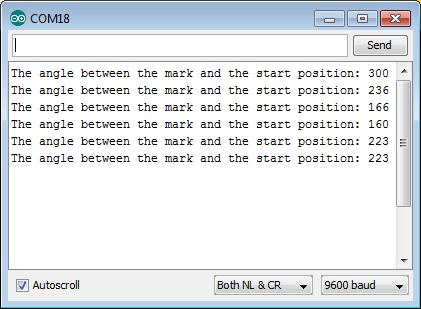# Measuring angle by potentiometer

In this example we will demonstrate how to use an analog potentiometer and how to display the reading in the Serial Monitor. This is another example of using the analog pins for input and using the serial connection to send data to a USB connected PC. Additionally, this example demonstrates the use of the board reference voltage for processing analog input signals into usable readings. In this case the angular reading expressed in degrees. This type of sensor can be used to measure and record the direction that the wind is blowing.

## Required Components• SODAQ Mbili Board
• 0.5W Solar Panel
• 1aH Battery Pack
• Grove Rotary Angle Sensor
• 1x Grove Cable (any length)

• none

## Hardware Setup

You should refer to both the board diagram and Grove sockets page for additional information.

1. First, plug the Rotary Angle Sensor into the socket for the analog pins A0 A1.
2. Then, plug the 0.5W solar panel and the 1A LiPo battery into their respective sockets.Turn on the SODAQ Mbili board, compile and upload the following sketch from the Arduino IDE onto the SODAQ Mbili board. Leave the USB cable plugged in and open the Serial Monitor (Ctrl-Shift-M) and ensure that it is set to the 9600 baud rate.

After opening the Serial Monitor (Ctrl-Shift-M), if you rotate the Rotary Angle Sensor you should see output similar to this:## Sketch Code

```#define ROTARY_ANGLE_SENSOR A0 //Use analog pin A0 for the Rotary Angle Sensor
#define FULL_ANGLEВ 300.0 //Full value of the rotary angle is 300 degrees

void setup()
{
//Start the serial connection
Serial.begin(9600);
}

void loop()
{
//Read the value of the rotary angle sensor in degrees
int degrees = getDegrees();

//Output it to the serial monitor
Serial.print("The angle between the mark and the start position: ");
Serial.println(degrees);

delay(500);
}

int getDegrees()
{

//Convert the sensor reading to degrees and return that value
float voltage = (float)sensor_value * ADC_REF / 1023;
float degrees = (voltage * FULL_ANGLE) / ADC_REF;
return degrees;
}
```

## Sketch Code Breakdown

### Globals

Here we specify the analog pin which we will connect to the Rotary Angle Sensor. Additionally, we specify several constants, including reference voltages for the ADC and the angular range of the sensor, which are used for converting the analog signal to a value in degrees.

```#define ROTARY_ANGLE_SENSORВ A0 //Use analog pin A0 for the Rotary Angle Sensor
#define FULL_ANGLEВ 300.0 //Full value of the rotary angle is 300 degrees
```

### setup()

Here we simply start the serial connection with a call to Serial.begin().

```void setup()
{
//Start the serial connection
Serial.begin(9600);
}
```

### loop()

Here we first get the reading from the sensor by calling the user defined method getDegrees(). This method returns the processed analog signal as an integer value in degrees. We then write data to the outgoing stream buffer of the serial connection using the Serial.print() & Serial.println() methods. This data includes a text description and the reading from the Rotary Angle Sensor in degrees.

```void loop()
{
//Read the value of the rotary angle sensor in degrees
int degrees = getDegrees();

//Output it to the serial monitor
Serial.print("The angle between the mark and the starting position: ");
Serial.println(degrees);

delay(500);
}
```

## getDegrees()

In this method the raw analog signal is read from the pin connected to the Rotary Angle Sensor. The reading is then processed and returned as a value representing the angle of the sensor in degrees.

First the raw signal is read from the pin using analogRead(). This is then converted into a voltage reading by multiplying it by the ADC_REF reference voltage and dividing it by the upper boundary of the reading’s range (1023). The result is a floating point value with a range of 0.0…ADC_REF. The final value, in degrees, is calculated by multiplying the reading voltage by the angular range of the sensor (FULL_ANGLE) and then dividing it by the ADC_REF reference voltage. The final value has a range of 0…FULL_ANGLE as is return by the method.

```int getDegrees()
{
// Read the raw sensor value